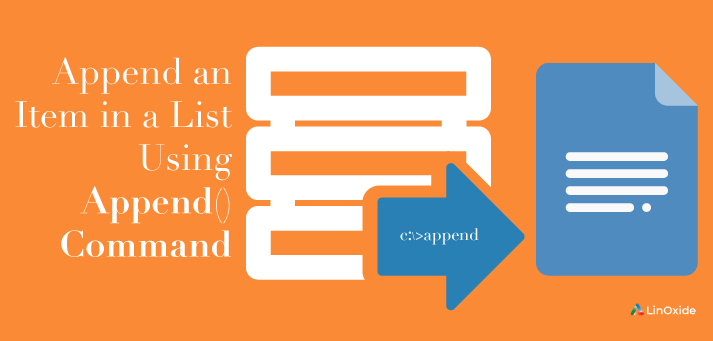We have a list of numbers or strings, and we want to append items to a list. Basically, we can use the `append` method to achieve what we want.

The `append()` method adds a single item to the existing list. It doesn’t return a new list; rather it modifies the original list.

The syntax of `append()` method

`list.append(item)`

append() Parameters

The `append()` method takes a single item and adds it to the end of the list. The item can be numbers, strings, another list, dictionary etc.

## 1)  Adding Element to a List

Let’s look adding element to a list with an example

```  # fruit list
fruit = ['orange', 'apple', 'banana']

fruit.append('pineapple')

#Updated Fruit List
print('Updated fruit list: ', fruit)
```
```  output
Updated animal list: ['orange', 'apple', 'banana', 'pineapple']
```

as you see , `'pineapple'` element has been added as last element.

## 2) Adding list to a list

Let’s see how to add list to a list

```  # fruit list
fruit = ['orange', 'apple', 'banana']

# another list of green fruit
green_fruit = ['green apple', 'watermelon']

# adding green_fruit list to fruit list
animal.append(green_fruit)

#Updated List
print('Updated animal list: ', fruit)
```
```  output
Updated animal list: ['orange', 'apple', 'banana', ['green apple', 'watermelon']]
```

As you see when append green_fruit list to fruit list, it goes as a list not two element.

## 3) Adding element of list to a list

Here we wil use `extend()` method to add element of list to another list, we will use the same pravious example to see the difference.

```  # fruit list
fruit = ['orange', 'apple', 'banana']

# another list of green fruit
green_fruit = ['green apple', 'watermelon']

# adding green_fruit list to fruit list
animal.extend(green_fruit)

#Updated List
print('Updated animal list: ', fruit)
```
```  output
Updated animal list: ['orange', 'apple', 'banana', 'green apple', 'watermelon']
```

As we saw, the green_fruit list has been added as elements not as a list to fruit list.

## 4) Adding element to a list with for loop

We will use `For loop` to append groups of element to list.

```  # numbers list
numbers = []

# use for loop to fill numbers list with elements
for i in range(10):
numbers.append(i)

#Updated List
print('Updated numbers list: ', numbers)
```
```  output
Updated numbers list:  [0, 1, 2, 3, 4, 5, 6, 7, 8, 9]
```

We made empty list `numbers` and used for loop to append numbers in range from 0 to 9, so for loop in frist working append 0 and check number 2 in range or not, if in range append it and so on until reaching number 9, which add it and for loop stop working.

## 5) Adding element to a list with while loop

We will use `While loop` to append groups of element to list.

```  # temperature list
temperature = []

# use while loop to fill temperature list with temperature degrees
degree_value = 20
degree_max = 50
while degree_value <= degree_max:
temperature.append(degree_value)
degree_value += 5

#Updated Temperature List
print('Updated temperature list: ', temperature)
```
```  output
Updated temperature list:  [20, 25, 30, 35, 40, 45, 50]
```

we made empty list `temperature` and put start point `degree_value` and limit point `degree_max` and said, while degree_value less than or equal degree_max, append this value to temperature list, after that increase the value of degree_value five degrees, while loop will work until degree_value equal degree_max and stop working.

## 6) Appending two array using numpy module

We will use `append` method in `numpy` module to append two arrays.

```  # import numpy module
import numpy as np

A = np.array()
B = np.array([1,5,5])
C = np.append(A, B)

#print array C
print('Array C: ', C)
```
```  output
Array C:  [3 1 5 5]
```

Note: you should install `numpy` module first by this command `\$ pip3 install numpy`.

### Conclusion

In this article, we have learned how to add an item in a list using Python append() command. Please let me know if you have any questions.